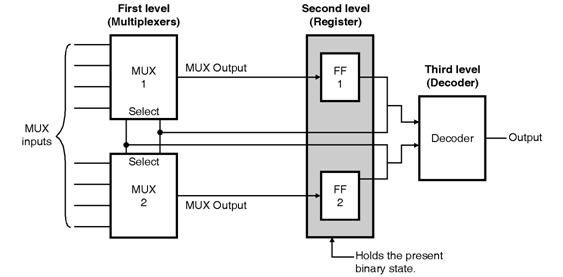Home > finite state machines > Algorithmic State Machines > Design with Multiplexers

# Design-with-Multiplexers

Design with Multiplexers :

• The first method of realizing a logic circuit from the ASM chart simplify using the K-map simplification. We have discussed this method in the preceding section.
• The other method of realization is by using the multiplexers. We will discuss this method in this section.
• A combinational circuit can be implemented with the help of multiplexers instead of individual gates. Hence we are going to replace the combinational circuit that uses gates by a multiplexer.
• The multiplexer method of design has three levels of components, as shown in Figure below.• The multiplexer outputs are applied to the flip flops forming the register at second level. The second level register is used for holding the present binary state.
• The multiplexers decide the next state of the register because multiplexer output has been connected to the flip-flop inputs as shown in Figure.
• The third level has a decoder which provides a separate output for each control state. Sometimes the decoder is replaced by a combinational circuit.
• The input to this decoder come from the outputs of the register flip flops and the inputs X1, X2 etc.
• In order to understand the design with multiplexers let us use the same waveform generator example.